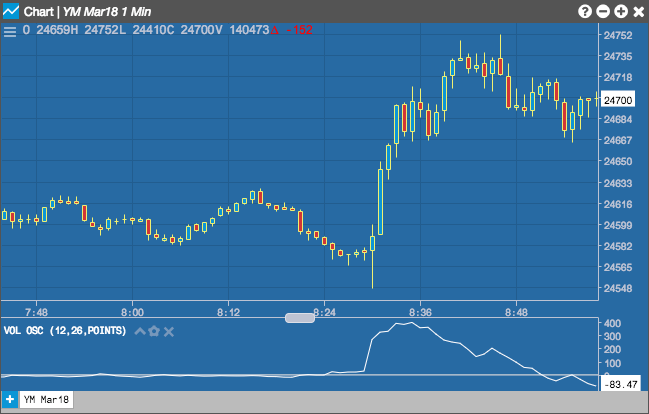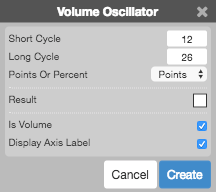Charts

# Volume Oscillator

The Volume Oscillator displays the difference between two moving averages of a security's volume.## Configuration Options• Short Cycle: Number of periods in the fast volume moving average.
• Long Cycle: Number of periods in the slow volume moving average.
• Points or Percent: Whether to display the curve as the actual difference or as a percentage of the fast volume moving average.
• Color Selectors: Colors to use for graph elements.
• Is Volume: TBD
• Display Axis Label: Whether to display the most recent value on the Y axis.

## Formula

$VO = \frac{Shorter Moving Average - Longer Moving Average}{Shorter Moving Average} \times 100$